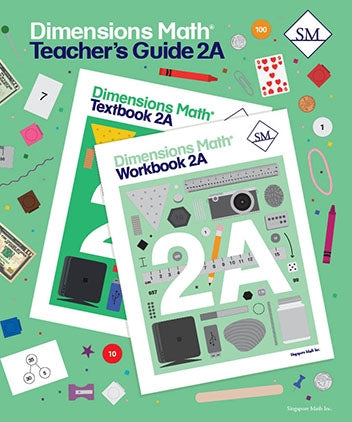# Dimensions Math Teacher's Guide 2A

Dimensions Math® Teacher's Guide 2A

Chapter 1: Numbers to 1,000

Teaching Notes
Chapter Opener
Lesson 1: Tens and Ones
Lesson 2: Counting by Tens or Ones
Lesson 3: Comparing Tens and Ones
Lesson 4: Hundreds, Tens, and Ones
Lesson 5: Place Value
Lesson 6: Comparing Hundreds, Tens, and Ones
Lesson 7: Counting by Hundreds, Tens, or Ones
Lesson 8: Practice
Workbook Solutions

Chapter 2: Addition and Subtraction — Part 1

Teaching Notes
Chapter Opener
Lesson 2: Strategies for Subtraction
Lesson 3: Parts and Whole
Lesson 4: Comparison
Lesson 5: Practice
Workbook Solutions

Chapter 3: Addition and Subtraction — Part 2

Teaching Notes
Chapter Opener
Lesson 2: Subtraction Without Regrouping
Lesson 3: Addition with Regrouping Ones
Lesson 4: Addition with Regrouping Tens
Lesson 5: Addition with Regrouping Tens and Ones
Lesson 6: Practice A
Lesson 7: Subtraction with Regrouping from Tens
Lesson 8: Subtraction with Regrouping from Hundreds
Lesson 9: Subtraction with Regrouping from Two Places
Lesson 10: Subtraction with Regrouping across Zeros
Lesson 11: Practice B
Lesson 12: Practice C
Workbook Solutions

Chapter 4: Length

Teaching Notes
Chapter Opener
Lesson 1: Centimeters
Lesson 2: Estimating Length in Centimeters
Lesson 3: Meters
Lesson 4: Estimating Length in Meters
Lesson 5: Inches
Lesson 6: Using Rulers
Lesson 7: Feet
Lesson 8: Practice
Workbook Solutions

Chapter 5: Weight

Teaching Notes
Chapter Opener
Lesson 1: Grams
Lesson 2: Kilograms
Lesson 3: Pounds
Lesson 4: Practice
Review 1
Workbook Solutions

Chapter 6: Multiplication and Division

Teaching Notes
Chapter Opener
Lesson 1: Multiplication — Part 1
Lesson 2: Multiplication — Part 2
Lesson 3: Practice A
Lesson 4: Division — Part 1
Lesson 5: Division — Part 2
Lesson 6: Multiplication and Division
Lesson 7: Practice B
Workbook Solutions

Chapter 7: Multiplication and Division of 2, 5, and 10

Teaching Notes
Chapter Opener
Lesson 1: The Multiplication Table of 5
Lesson 2: Multiplication Facts of 5
Lesson 3: Practice A
Lesson 4: The Multiplication Table of 2
Lesson 5: Multiplication Facts of 2
Lesson 6: Practice B
Lesson 7: The Multiplication Table of 10
Lesson 8: Dividing by 2
Lesson 9: Dividing by 5 and 10
Lesson 10: Practice C
Lesson 11: Word Problems
Review 2
Workbook Solutions

Blackline Masters for 2A

Dimensions Math® PreK-5 series features the progression, rigor, and pacing that define Singapore math. Throughout the series, five characters offer students suggestions on how to think about problems. They remind students of strategies they’ve learned and point out important information that encourages them to come up with their own solutions.

Textbook lessons begin with a task that allows students to apply their previous knowledge and learn through discussion. Once students have mastered a concept with the use of concrete and pictorial aids, they are ready to take on more abstract mathematical problem sets. They reach fluency by collecting various strategies along the way and applying them to new problems. Word problems give students a sense of math in real-world contexts.

Workbooks offer independent practice that follows a careful progression of exercise variation. Each textbook lesson includes a corresponding workbook exercise that starts with pictorial representation and progresses to more challenging abstract problems. Workbooks for PreK-2 are perforated.

Teacher’s Guides include lesson plans, mathematical background, games, helpful suggestions, and comprehensive resources for daily lessons. Lessons are laid out clearly and activities are designed for the whole class, small groups, and extension.

Textbooks and Workbooks do not include answer keys. Answers are in Teacher's Guides.
ISBN  9781947226340